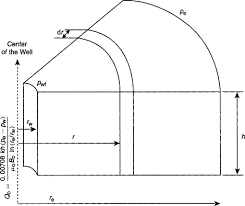## How to Calculate and Solve for Radial Flow Rate in Reservoir Fluid Flow | The Calculator EncyclopediaThe image above represents the radial flow rate.

To compute for the radial flow rate, eight essential parameters are needed and these parameters are External Pressure (Pe), Flowing Bottom-Hole Pressure (Pwf), Formation Thickness (h), Oil Viscosity (μo ), Permeability (k), Oil Formation Volume Factor (Bo), Drainage Radius (reand Well Bore Radius (rw ).

The formula for calculating the radial flow rate:

Qo = 0.00708kh[Pe – Pwf] / μo Bo In[re / rw]

Where;

Qo = Radial Flow Rate
Pe = External Pressure
Pwf = Flowing Bottom-Hole Pressure
h = Formation Thickness
μo = Oil Viscosity
k = Permeability
Bo = Oil Formation Volume Factor
re = Drainage Radius
rw = Well Bore Radius

Let’s solve an example;
Find the radial flow rate when the External Pressure is 14, Flowing Bottom-Hole Pressure is 21, Formation Thickness is 7, Oil Viscosity is 35, Permeability is 50, Oil Formation Volume Factor is 13, Drainage Radius is 26 and Well Bore Radius is 15.

This implies that;

Pe = External Pressure = 14
Pwf = Flowing Bottom-Hole Pressure = 21
h = Formation Thickness = 7
μo = Oil Viscosity = 35
k = Permeability = 50
Bo = Oil Formation Volume Factor = 13
re = Drainage Radius = 26
rw = Well Bore Radius = 15

Qo = 0.00708kh[Pe – Pwf] / μo Bo In[re / rw]
Qo = 0.00708 x 50 x 7 [14 – 21] / 35 x 13 In[26 / 15]
Qo = 0.00708 x 50 x 7 [-7] / 35 x 13 In[26 / 15]
Qo = 0.00708 x 50 x 7 [-7] / 35 x 13 In[1.73]
Qo = 0.00708 x 50 x 7 [-7] /35 x 13 x 0.55
Qo = 2.478 [-7] / 35 x 13 x 0.55
Qo = -17.346 / 250.27
Qo = -0.069

Therefore, the radial flow rate is -0.069 STB/day.